# Parallel Courses III

## Introduction

A graph is a data structure that has nodes containing the required information. We call these nodes vertices. A vertex is connected to another vertex through an edge.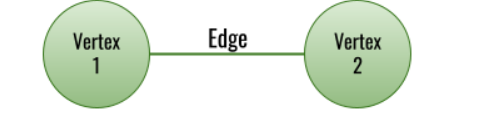An edge can be one-way or two-way. One-way edges create directed graphs, while two-way edges create undirected graphs.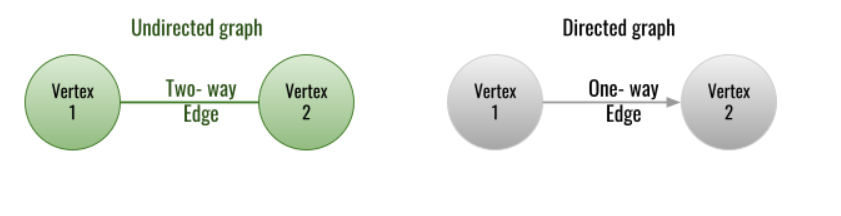To represent a directed or undirected graph, we use two types of data structure:

• Adjacency Matrix: An adjacency matrix is a 2-D binary matrix with values either true or false. An adjacency matrix has a number of columns and rows equal to the number of vertices in the graph.

• Adjacency list: As the name suggests, an adjacency list stores only those vertices in a list adjacent to the given vertex. An adjacency list is a 2-D vector with the number of rows equal to the number of vertices, with each row containing the list of adjacent vertices.

Before we move on to the problem statement, we must know the concept of topological sorting. Topological sorting refers to the sorting of graph vertices in a way that one vertex comes before the other due to a particular criterion. Only directed acyclic graphs can be sorted topologically. A directed acyclic graph is one that does not contain any cycle in any possible path of the vertices. Topological sorting is done to resolve the dependencies. That means if there are ‘N’ number of vertices named as V1,  V2, V3, and so on and a vertex ‘Vi’  has to be taken care of before another vertex ‘Vj’, a topological sorting algorithm will provide a sequence of vertices in which vertex ‘Vi’  is attended before another vertex ‘Vj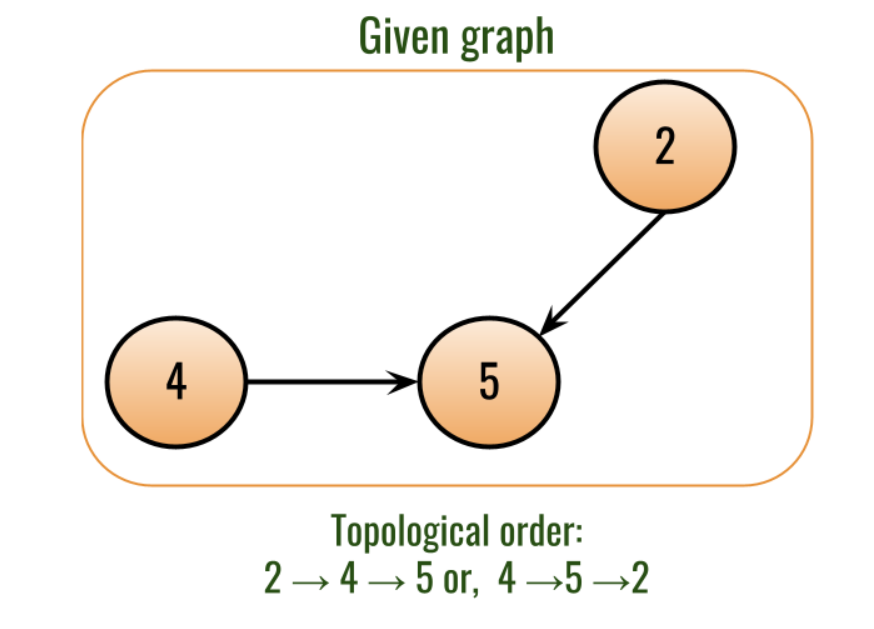## Problem Statement

directed acyclic graph where each vertex represents a course and an edge from vertex Vto Vrepresenting that course Vis a prerequisite for course Vis given. Also, an array ‘TIME’ is given, representing the number of months taken to complete a particular course. Find the minimum number of months required to complete the parallel courses if the rules given below are followed:

• You can start a course at any time if all prerequisite courses are finished.
• Any number of parallel courses can be started at the same time.

Note that the given parallel courses are 1-indexed while the time array is 0-indexed.

### Explanation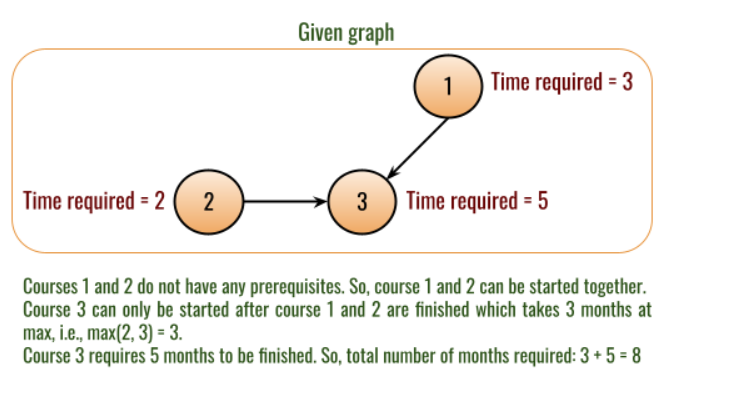## Approach

Before moving on to the approach, we need to transform the input into a standard data structure. So, we’ll convert the given input of the graph into an adjacency list.

The vertices of the graph are dependent on the other vertices. We know that Topological sorting is done to resolve the dependencies. So, we can use topological sorting to solve the problem.

In the Explanation part of the Problem Statement section, you may have noticed that Course 3 has two parent vertices - Course 1 and Course 2. Out of these two vertices, we included Course 1’s time in the answer. This is because Course 2 might be ended after 2 months, but Course 3 can not start until both the parent parallel courses are finished. You can refer to the timeline from the image given below: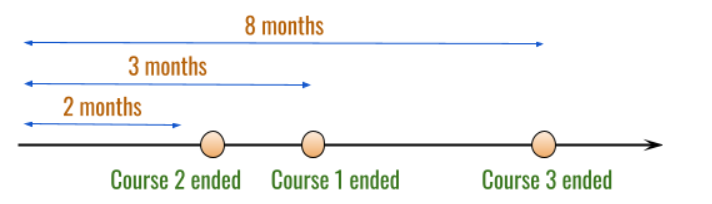Use a vector ‘IN_DEGREE’ to store the number of parents of a vertex left unprocessed. So, if ‘IN_DEGREE’ is equal to 0, that means all the parents of the current vertex are processed. Only when a vertex has the  ‘IN_DEGREE’ equal to 0, it will be processed.

To do the topological sorting, we will use a queue that stores pairs of vertex numbers (Course number) and its respective number of unexplored parents of the course (‘IN_DEGREE’).

We will create a vector 'C_TIME' to save the final completion time for all the parallel  courses.  If the current vertex is completed at the time ‘T’, then all its child vertices would be completed at the time ‘T’+ TIME[i], where TIME[i] represents the completion time of the child vertex. While pushing the children vertices to the queue, change their completion time accordingly.

After processing all the vertices, the maximum completion time of all the vertices would be considered the minimum number of months required to complete all the parallel courses. Refer to the algorithm given below for a better understanding.

## Algorithm

• Create a queue ‘Q’ of type pairs of integers. (Pair in queue represent Course number and number of unexplored parents of the course).
• Push all the vertices with zero number of parents into the queue.
• Create a vector ‘C_TIME’ representing the final completion of the courses.
• Initialize the ‘MAX_COMPLETION_TIME’ variable to store maximum completion time of all the vertices.
• While ‘Q’ is not empty, do:
• Initialize ‘FRONT_PAIR’ equal to the front pair of the queue ‘Q’.
• Pop the front pair from the queue.
• Initialize ‘NODE’ equal to the first element of the ‘FRONT_PAIR’.
• Initialize ‘T’ equal to the second element of the ‘FRONT_PAIR’.
• Initialize COMPLETION_TIME = T + TIME[NODE].
• Set MAX_COMPLETION_TIME = max(MAX_COMPLETION_TIME,  COMPLETION_TIME).
• C_TIME[NODE] = COMPLETION_TIME .
• Iterate the adjacency list of  ‘NODE’ using ‘CHILD’ variable(to change the completion time of the children vertices), do:
• Set C_TIME[CHILD] = max(C_TIME[CHILD], COMPLETION_TIME ).
• Decrement IN_DEGREE[CHILD]. (to indicate the removal of current parent dependency).
• If IN_DEGREE[CHILD] equal to 0 (All the parents are explored), then:
• Push {CHILD, C_TIME[CHILD]} pair to the queue ‘Q’.
• Return ‘MAX_COMPLETION_TIME’.

## Program

``````#include <iostream>
#include <vector>
#include <queue>
using namespace std;

int minimumTime(int numCourses, vector<vector<int>> &edges, vector<int> &time)
{

// To represent graph as an adjacency list.

// To represent number of parents unexplored for the current vertex.
vector<int> inDegree(numCourses);

// To represent completion time for each vertex.
vector<int> cTime(numCourses, 0);

// Convert the given input of the graph into an adjacency list.
for (vector<int> edge : edges)
{
int edgeStart = edge - 1;
int edgeEnd = edge - 1;
inDegree[edgeEnd]++;
}

queue<pair<int, int>> q;

// Push all the vertices with zero number of parents into the queue.
for (int i = 0; i < numCourses; i++)
{
if (inDegree[i] == 0)
{
q.push({i, 0});
}
}

int maxCompletionTime = 0;
while (!q.empty())
{
pair<int, int> frontPair = q.front();
q.pop();

int node = frontPair.first;
int t = frontPair.second;

// Completion time for current vertex.
int completionTime = t + time[node];

// Comparing completion time for current vertex with completion time of all the vertices so far.
maxCompletionTime = max(completionTime, maxCompletionTime);
cTime[node] = completionTime;

{

// Maximum completion time for child so far.
cTime[child] = max(cTime[child], completionTime);

// To indicate the removal of current parent dependency.
inDegree[child]--;

// If all the parents are explored, push child into queue to explore child vertex.
if (inDegree[child] == 0)
{
q.push({child, cTime[child]});
}
}
}
return maxCompletionTime;
}

int main()
{

// Input number of courses given.
int numCourses;
cout << "Enter the number of vertices in the graph: ";
cin >> numCourses;
cout << "Enter edges as U V representing an edge from vertex U to vertex  V.\n";
cout << "Enter -1 -1 to stop.\n";

// 'EDGES' to store the graph in given format.
vector<vector<int>> edges;
while (true)
{
int u, v;
cin >> u >> v;
if (u == -1 and v == -1)
break;
edges.push_back({u, v});
}

cout << "Enter the completion time for each course: ";
vector<int> time(numCourses);
for (int i = 0; i < numCourses; i++)
cin >> time[i];
cout << "Minimum number of months required to complete all the courses: ";
cout << minimumTime(numCourses, edges, time);
}
``````

## Complexity Analysis

Time complexity: O(N+E). Where ‘N is the number of nodes, and ‘E’ is the number of edges.
Space complexity: O(N+E). Where ‘N’ is the number of nodes, and ‘E’ is the number of edges.

## Key Takeaways

Congratulations on your learning! Parallel Courses III is an interesting question, but it is not the only interesting question here. Find more interesting questions on our practice platform CodeStudio. If you want to learn more before jumping into practicing, head over to our library section for many such interesting blogs. Keep learning.

Happy Coding!